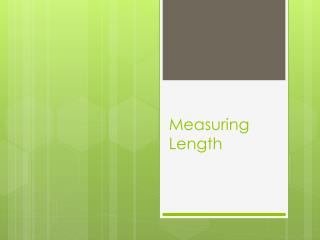DownloadDownload PresentationMeasuring Length

# Measuring Length

Télécharger la présentation## Measuring Length

- - - - - - - - - - - - - - - - - - - - - - - - - - - E N D - - - - - - - - - - - - - - - - - - - - - - - - - - -
##### Presentation Transcript

1. Measuring Length

2. Length Units • The main unit for length is the meter or “cm” for short • There are also other smaller and bigger units like: • Millimeters or “mm” for short • Centimeters or “cm” for short • Kilometers or “km” for short • The units are how we label an answer

3. Length Tools/Instruments • The tool or instrument used to find length is either a ruler or a meterstick

4. Two sides of a Ruler • There are two sides to a ruler: centimeters and inches • In science you will NEVER EVER use the inches side! • The smaller/shorter dashes with NO numbers represent millimeters “mm” • The longer dashes with numbers represent centimeters “cm”

5. Finding the Length • Find the twobig numbers that the line/object is between. • Use the lower of the two numbers. This is the first part of your answer. • Now count the dashes after the lower number. This is the second part of your answer • Take both parts of your answer and put a decimal between the two numbers • Label your answer with the unit centimeters or “cm” for short.

6. The nail is between ___5______ and ____6_____ • I will use the _____5____ since it is smaller • 3. I count ______1_______ dashes after the big number • Answer: ____5___.____1___ centimeters

7. The lineis between ____3_____ and _____4____ • 2. I will use the ____3_____ since it is smaller • 3. I count _____4________ dashes after the big number • 4. Answer: ____3___.___4____ centimeters

8. The lineis between _________ and _________ • 2. I will use the _________ since it is smaller • 3. I count _____________ dashes after the big number • 4. Answer: _______._______ centimeters

9. The lineis between ____11_____ and ____12_____ • 2. I will use the ___11______ since it is smaller • 3. I count ______6_______ dashes after the big number • 4. Answer: ___11____.___6____ centimeters

10. The lineis between _________ and _________ • 2. I will use the _________ since it is smaller • 3. I count _____________ dashes after the big number • 4. Answer: _______._______ centimeters

11. The lineis between ____1_____ and ____2_____ • 2. I will use the ___1______ since it is smaller • 3. I count ______8_______ dashes after the big number • 4. Answer: __1_____._____8__ centimeters